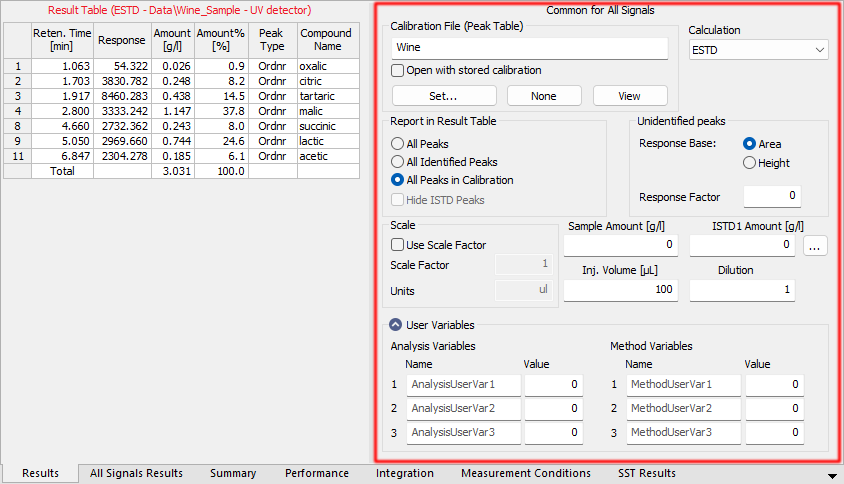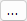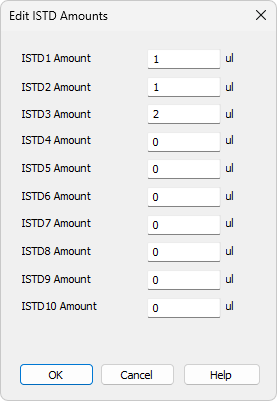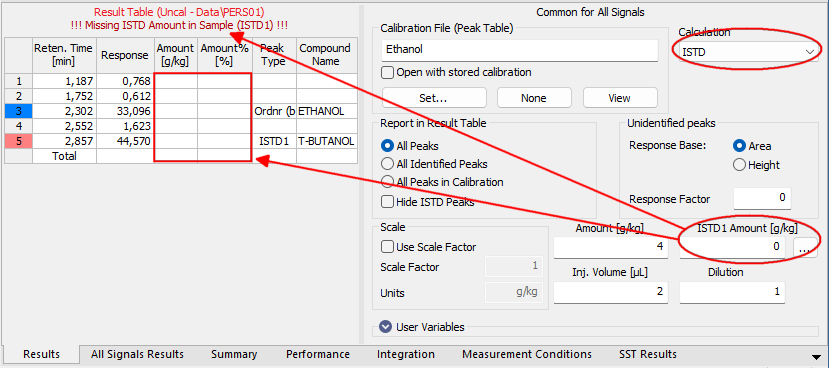# Calculation

Selects the method for calculating the results of the chromatogram. Once selected, the Results tab will be displayed in the lower part of the Chromatogram window. In its right-hand section it is possible to define the method of results calculation. Left-hand section displays Result table with signal related results.

The parameters stated here are common for all signals when a multi-detector chromatogram is being used. The displayed values come from the template method; they are filled in on the Calculation tab of the Method Setup dialog as a part of the method according which the chromatogram is measured.

Calibration File (Peak Table)

Name of the calibration file used to obtain calibrated calculations.

Open with stored calibration

When checked, the chromatogram will be opened with calibration stored within the chromatogram file when it is opened next time. To open the chromatogram with the stored calibration this way it is necessary to re-open the chromatogram using the Open Chromatogram dialog - re-opening the Chromatogram window is not sufficient. Similar behavior may be set directly when the chromatogram is created from Sequence window or set in the Single Analysis - Post Run Settings tab.

Note:

The differences between Stored and Linked calibration are explained in the Open Chromatogram window in the Method section.

Set…

Invokes the Open Calibration dialog where the calibration file may be selected.

None

Closes the opened calibration file and sets the calculation type in the Calculation field to Uncal (uncalibrated).

View

Opens the linked calibration file in the Calibration window. Current chromatogram will be automatically opened in it, ready for adding new levels.Chromatogram - Results tab - Results

Calculation

Selects the type of calculation. Available options are shown Tab "List of available calculation types".

List of available calculation types

Calculation type Way of calculation
Uncal - Uncalibrated calculation without use of calibration file. The result of the integration is a percentage representation of individual areas and heights of peaks in the chromatogram. When using this option, all other sections on this tab will be inactive and dimmed.
ESTD - External standard calculation. The result will be both a percentage and absolute representation of individual substances and their names.
ISTD - Internal standard calculation. The result will be both a percentage and an absolute representation of individual substances and their names.
NORM - External standard calculation with add-on checks. The result will be both a percentage and an absolute representation of individual substances and their names. In case any peak found in the Result Table is not present in a calibration and/or the Amount parameter differs from zero, the calculation of the Peak Type for all rows in the Result Table will be Error and the Amount [%] values will not be calculated.
STDADD - Standard Addition calculation. The result amounts of compounds in an unknown sample are calculated based on standard addition calibration curve by extrapolation of the calibration curve to point with Y coordinate equal to zero. The results are displayed in absolute values.

Note:

If only the Peak Type field for selected rows of the Result Table show the Error code, than the corresponding compounds do not have the Amount value filled in the calibration file.

Report in Result Table

This field determines which peaks will be displayed in the Result Table and included in the sum for the Total row in the case of calibrated calculations.

All Peaks

All integrated peaks in the chromatogram will be displayed.

All Identified Peaks

Only peaks identified in the calibration file will be displayed.

All Peaks in Calibration

All peaks from the calibration file will be displayed without regard to how many peaks the chromatogram contains and how many were identified. This provides what is known as a fixed table format given by the calculation of peaks in the calibration file.

Hide ISTD Peaks

The peaks identified as the internal standards will not be displayed in the Result Table.

Note:

The command will be active only when the ISTD calculation type has been selected.

Unidentified Peaks

Response Base

Selects a base for calibrated calculation of unidentified substances.

Area - Calculations will be derived from peak areas.

Height - Calculations will be derived from peak heights.

Response Factor

Coefficient used for amount calculation for unidentified substances. Amount is set as area (height) of peak multiplied by this coefficient. This value serves in fact as an artificial single-point calibration for unidentified substances.

Scale

Area for entering the auxiliary calculation parameters.

Use Scale Factor

The whole area is activated by checking this checkbox.

Scale Factor

All values of the Amount column in the Result Table will be multiplied by the stated value.

Units

Units for application of scale. The original units taken from the calibration file are replaced by the units defined here in the results table.

Amount

Amount of the sample. This value is used as a basis for the calibrated calculation of the percentage representation of individual substances for ESTD and ISTD methods.

When the ISTD method is used, enter the amount of the sample without the internal standard. State the parameter in units used in the calibration file (Units - Compound parameter in the Calibration Options dialog). The implicit value is 0. In this case the percentage representation is calculated from the Amount sum in the Total row.

Note:

If the Amount parameter is set to zero, the percentage representation of individual values will be calculated from the sum of all calibrated amounts (including substances calibrated using the Uncal. Response parameter). Otherwise the calculation is based on the Amount value entered.

ISTD Amount

Specifies the amount of internal standard (ISTD1) added when the results are calculated using the ISTD method. Similar settings are available for the ISTD2 - ISTD10 in the Edit ISTD Amounts dialog accessible through thebutton.Edit ISTD Amounts

Edit ISTD Amounts dialog allows to set the ISTD Amounts for all internal standards used in particular chromatogram. It is also accessible from the Single Analysis dialog and amounts can be entered from the Sequence window (settings in the ISTD1 Amount - ISTD10 Amount columns, only ISTD1 Amount column is displayed by default). Enter the parameters in units used in the calibration file (Units - Compound parameter in the Calibration Options dialog). The implicit value is 0. If the same amount of the internal standard is used in all used calibration standards and unknown samples, this amount need not be entered and can be left at 0 in both calibration and chromatograms (sequence).

Caution:

When the amount of the internal standard is entered either in the calibration file (Amount column) only or in the unknown sample (ISTD Amount parameter) only and zero will be left in the other place, the Result Table will not display any results and the header will show an inscription stating the cause of the problem. The compound will be also omitted from the Summary Table.Chromatogram - Calculation - ISTD settings

Inj. Volume

Specifies the size of the injection. The response of all substances calculated using the calibrated calculation is corrected by the ratio of this value to the value of Default Injected Volume field set in the Calibration Options dialog. Inj. Volume parameter will influence the calculation only after the Default Injected Volume value is set. The implicit value is 0.

Note:

The Inj. Volume parameter can be applied for injected amount correction while using autosamplers unable to determine precisely the quantity to be injected, but fully capable to determine (ex-post) the quantity which has been injected.

Dilution

Specifies the dilution of the sample. It is the total number of unit volumes in which the sample was dissolved. In other words, dilution represents the total volume of solute + solvent. Each calibrated amount in the Amount column of the Result Table is multiplied by this value.The implicit value is 1.

User Variables

Display and enable to edit Name and Value of User Variables used in User Columns calculations performed in Result Table.

Analysis Variables

Specifies up to three Analysis User Variables. Analysis User Variables are editable through Single Analysis dialog, Sequence window or directly in Chromatogram window at Results tab and All Signals Results tab.

Method Variables

Specifies up to three Method User Variables. Method User Variables are editable through Method Setup dialog at Advanced tab or directly in Chromatogram window at Results tab and All Signals Results tab.